# Is the kinetic energy dependent on the mass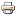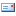This physics tutorial shows how to calculate kinetic energy *. The prerequisite for this is a basic knowledge of the term energy.
In the following, the term kinetic energy should be explained before the calculation continues.

### What is kinetic energy?

The kinetic energy Ekin, also called kinetic energy, is a measure of how much work has to be done on a body in order to bring it from rest to a certain state of motion. It is influenced by the mass m and the speed v of this body.

#####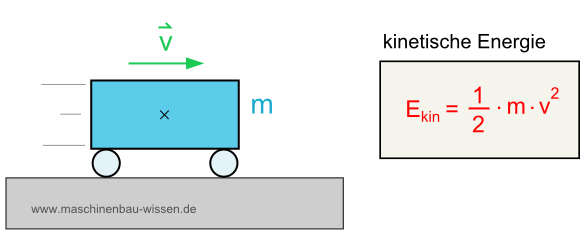Calculation of the kinetic energy of a mass m with the speed v

The kinetic energy is a state variable. This means that it depends exclusively on the current state of the system under consideration and is therefore completely independent of the path to reach this state.

The kinetic energy is inherent in the state of motion of a body. It changes proportionally to the square of the velocity v and to the mass m, measured on the inertial system in which the body is described.

As a result, the amount of kinetic energy depends on the point of view from which the system is viewed. Often an inertial system is used for this, which rests in relation to the ground.

Formula symbol: Ekin

Unit: J (Joule) = Nm (Newton meter) = kg m2· S-2

### Formula for calculating the kinetic energy

The speed v is related to the respective reference system. The driver of a moving car has a kinetic energy of E compared to his vehicle (= reference system)kin = 0 J. If, on the other hand, the road is used as a reference system when calculating kinetic energy, both the driver and the car have kinetic energy Ekin > 0 y.

### Calculate kinetic energy - formula:The dependence of the kinetic energy on the square of the speed v plays a role that should not be underestimated, among other things in car accidents. So doubling the speed v also means quadrupling the kinetic energy Ekin.

### Kinetic energy of rotating bodies

Extended bodies can perform not only a translational movement, but also a rotary movement. The kinetic energy contained in the rotational movement is called rotational energy. This is proportional to the body's moment of inertia and the square of the angular velocity.

### Calculate kinetic energy - example:

Now we come to a small task to calculate the kinetic energy.

A car with a mass m = 1000 kg drives at a speed v = 50 km / h. How big is the kinetic energy Ekin of the vehicle opposite the road? The equation for calculating the kinetic energy Ekin reads:

In order to calculate the kinetic energy, the speed v = 50 km / h must first be converted into meters per second (SI unit of speed).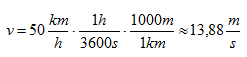Now, to calculate the kinetic energy Ekin the speed v and the mass m are inserted into the formula.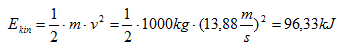Result:

The kinetic energy Ekin for a car traveling at 50 km / h across the street is 96465 J = 96.47 kJ.

What kinetic energy is and how one can calculate the kinetic energy * should now be clear to everyone. Other tasks on the topic can of course be much more complex, but the basic knowledge is a prerequisite for coping with tasks with a higher degree of difficulty.